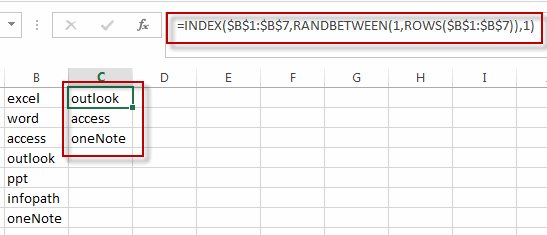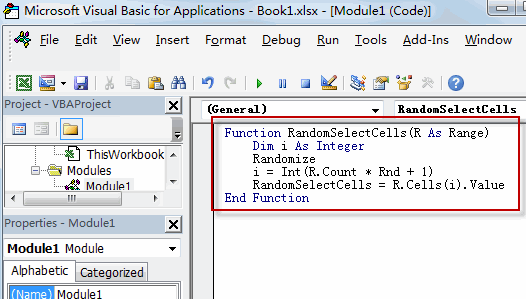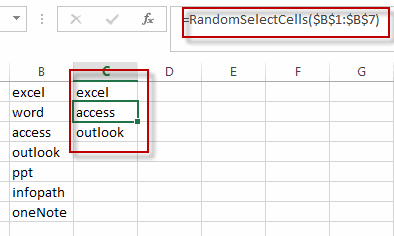# Randomly Select Cells

This post will guide you how to randomly select cells from a list or table in Excel. How to get random value form a range of cells in an Excel Spreadsheet.

Assuming that you have a list of data (B1:B7) in a worksheet, and you want to get 3 random cells or values from those data, how to achieve it. This post will introduce two method to get random value from a range of cells. Randomly Select Cells with Formula. Or how to use an Excel User Defined Function to select cells randomly.

## Randomly Select Cells with a Formula

If you want to randomly select cells from a range of cells, you can use a formula based on the INDEX function, the RANDBETWEEN function and the Rows function. Like this:

`=INDEX(\$B\$1:\$B:\$7,RANDBETWEEN(1,ROWS(\$B\$1:\$B:\$7)),1)`

Type this formula into a blank cell C1, and press Enter key in your keyboard, and then drag the AutoFill Handle over other two cells to apply this formula to get 3 random cells.## Randomly Select Cells with a User Defined Function

You can also write an User Defined Function with VBA code to quickly select random cells from a range of cells in Excel. Just do the following steps:

#1 open your excel workbook and then click on “Visual Basic” command under DEVELOPER Tab, or just press “ALT+F11” shortcut.#2 then the “Visual Basic Editor” window will appear.

#3 click “Insert” ->”Module” to create a new module.#4 paste the below VBA code into the code window. Then clicking “Save” button.```Function RandomSelectCells(R As Range)
Dim i As Integer
Randomize
i = Int(R.Count * Rnd + 1)
RandomSelectCells = R.Cells(i).Value
End Function```

#5 back to the current worksheet, try to enter the below formula in Cell C1.

`=RandomSelectCells(\$B\$1:\$B\$7)`### Related Functions

• Excel INDEX function
The Excel INDEX function returns a value from a table based on the index (row number and column number)The INDEX function is a build-in function in Microsoft Excel and it is categorized as a Lookup and Reference Function.The syntax of the INDEX function is as below:= INDEX (array, row_num,[column_num])…
• Excel RANDBETWEEN Function
The Excel RANDBETWEEN function returns a random integer number that is between the numbers you specify.The syntax of the RANDBETWEEN function is as below:= RANDBETWEEN (bottom,top)….
• Excel ROWS function
The Excel ROWS function returns the number of rows in a cell reference.The syntax of the ROWS function is as below:= ROWS(array)…